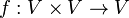# Algebraic group

This article gives a basic definition in the following area: algebraic group theory
View other basic definitions in algebraic group theory |View terms related to algebraic group theory |View facts related to algebraic group theory

This article describes a compatible combination of two structures: group and algebraic variety

This article defines the notion of group object in the category of algebraic varietys|View other types of group objects
This is a variation of group|Find other variations of group | Read a survey article on varying group

## Definition

### Abstract definition

An algebraic group or group variety over a field$K$ is a group object in the category of algebraic varieties over$K$.

### Concrete definition

An algebraic group or group variety over a field$K$ is a set$G$ equipped with the following two structures:

• The structure of an algebraic variety over the field$K$.
• The structure of a group

such that the following compatibility conditions are satisfied:

Multiplication or product 2 (so it's a map$G \times G \to G$) regular map from$G \times G$ (equipped with the variety structure on the product variety) to$G$. Note that the map being a regular map forces it to be continuous from$G \times G$ with its Zariski topology to$G$ with its Zariski topology. However, the Zariski topology on$G \times G$ is not the same as the product topology arising from the Zariski topology on$G$. This is an important part of the reason why algebraic groups fail to be topological groups.
On the other hand, it is true that the left and right multiplication maps by elements of$G$ are also regular morphisms, and hence are continuous as maps from$G$ to itself with the Zariski topology.
Identity element 0 (it's a constant element$e \in G$) no condition. As such, we may impose the condition that the map from a one-point variety to$G$ sending the point to the identity element is a regular morphism, but this condition is vacuously true.
Inverse map 1 (so it's a map$G \to G$) regular map as a map from$G$ to itself. Note that since the inverse map is its own inverse (see inverse map is involutive), this is equivalent to assuming that it is an isomorphism from$G$ to itself as an algebraic variety.

### Variation using separately instead of jointly algebraic

Further information: Separately algebraic group equals algebraic group

For an algebraic variety$V$, a map$f: V \times V \to V$ is termed separately algebraic if, for any fixed$v \in V$, the maps$x \mapsto f(x,v)$ and$x \mapsto f(v,x)$ are both regular morphisms from$V$ to itself. This is in contrast with jointly algebraic, which means regular from$V \times V$ with the product variety structure to$V$.

For infinite countable fields, it is possible to construct separately algebraic maps that are not jointly algebraic, even in the case where the variety$V$ is the field itself. Thus, a priori, we could define a separately algebraic version of the algebraic group definition where we require the group multiplication to only be separately algebraic, while still requiring the inverse map to be continuous. However, it turns out that any separately algebraic group must be algebraic.

However, we do obtain separate notions of left-algebraic group and right-algebraic group, where we assume algebraicity in only one of the two coordinates.

### Note on algebraic closure assumptions

The definition of algebraic group makes sense over any field. However, because many of the foundational results of algebraic geometry are valid only for algebraically closed fields, most basic treatments of algebraic groups consider algebraic groups over algebraically closed fields. To study what happens over a non-algebraically closed field, we typically put it inside its algebraic closure, then consider the algebraic group over the algebraic closure, and, if the formulas defining the group operations are all realized over the subfield, we can look at the points of the algebraic group corresponding to that subfield. For instance, to study elliptic curve groups over$\mathbb{Q}$, we consider the corresponding elliptic curve group over$\mathbb{C}$, then consider the subgroup of rational points inside that elliptic curve.

## Associated constructs

### Topological structure

The algebraic variety underlying an algebraic group has a topology. With this topology, it becomes a quasitopological group but almost never becomes a topological group. The reason is that the multiplication map is not jointly continuous with respect to the product topology on the product. This is related to the fact that the product topology on the product is considerably coarser than the Zariski topology on the product. An algebraic group is a quasitopological group because the multiplication map is separately continuous and the inverse map is continuous.

Moreover, in the finite-dimensional case, the quasitopological group is compact, sober, and T1 (hence, a T0 quasitopological group). See:

### Topological and analytic structure for some fields

In case the underlying field is a topological field, algebraic groups do become topological groups, not with respect to the Zariski topology, but with respect to a topology arising from the topology of the field. In fact, for fields that have an analytic structure, such as the field of real numbers, field of complex numbers, and various extensions of the p-adics, we get an analytic structure on the algebraic group, which is called a Lie group structure.

## Facts

### Linear algebraic groups and abelian varieties

There are two extreme cases of algebraic groups over algebraically closed fields: linear algebraic groups and abelian varieties. Every algebraic group can be broken down in terms of groups of these sorts:

## Relation with Lie group structure

For fields such as the real and complex numbers that have an analytic structure, algebraic groups are Lie groups. Some facts: